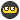## Recommended Posts

Hi all, I've been given a problem and am haiving a hell of approblem trying to figure it out.

In the following grid:I need to get all permetations of 4 moves. I can only move left,right,up or down, NOT diagonally. I have already stored all possible moves from each square in an array numbered 1 to 36.

So, say 1 start at square 1:

Available moves are 2,7

Loop through available moves

Get next moves (if 2 then 3,8 since 1 has already been used; if 7 then 8,13 since 1 has already been used)

Loop through available moves

...

...

Endloop

I would like to, and reckon this should be done with recursion.

Can anyone give me any pointers how to get going?

----[ SandyD ]---

##### Share on other sites

I need to get all permetations of 4 moves.

Is that exactly 4 moves, or 4 moves or less ? Notice that if you mean exactly that, when you start at square 1 you can't reach, for example, the squares 2 and 7.

And what do you mean with "permutations" ? Do you need a list of all "roads", or do you only want to know which numbers are reachable ?

I would like to, and reckon this should be done with recursion.

Depending on what you actually want a four simple "for" loops (inside each other) might do the trick too.##### Share on other sites

No not exactly, I can have 3 or 4 moves.

I need to know the path taken

In fact its actually up to 10 moves, so recursion is probably needed

----[ SandyD ]---

##### Share on other sites

Yes, you will probably need something like a \$CurrentGrid = [x,y] variable to start with. Then you need a list of all the values that you have already been through. Check the value of the currentgrid against the list. If it's not in the list, then add that grid to a array called \$GridsToCheck or something. Then \$CurrentGrid is read from \$GridsToCheck, and the cycle starts again.

Note: This is very much doable, so don't tell me you don't understand or can't make this on your own, cause I'm not going to do it for you. (I've had bad experiences with this)

##### Share on other sites

No not exactly, I can have 3 or 4 moves.

Thats, together with your last line ("actually up to 10 moves") a bit vague : why not 1 or 2 steps too ? And if 10 steps is your goal, how many steps are than valid ? 9 and 10 ? Or anything from 3 to 10 steps ?In fact its actually up to 10 moves, so recursion is probably needed

Nope. To proove that I included something that uses a simple X-by-3 array to remember previous X,Y and direction :
```;-- Storage for the steps & max path-length
dim \$path  ;-- The 4 here is the number of steps.  change if you like

;-- Width & height of field
\$w=10
\$h=10

\$i=0
;-- Starting-point
\$path[\$i]=8
\$path[\$i]=9
\$path[\$i]=0
do
if \$path[\$i]>=4 Then     ;-- All directions done ?
\$i-=1               ;-- Yep, back to previous step
else
;-- Where is the next location next of the current step ?
switch \$path[\$i]
case 0
\$x=\$path[\$i]+1
\$y=\$path[\$i]
case 1
\$x=\$path[\$i]
\$y=\$path[\$i]+1
case 2
\$x=\$path[\$i]-1
\$y=\$path[\$i]
case 3
\$x=\$path[\$i]
\$y=\$path[\$i]-1
EndSwitch
\$path[\$i]+=1         ;-- Increment current direction
if \$x>=0 and \$x<\$w and \$y>=0 and \$y<\$h then     ;-- Only if the next step is within the field
;-- Did we allready pass that next step ?
for \$j=0 to \$i-1
if \$x=\$path[\$j] and \$y=\$path[\$j] then ExitLoop
Next
if \$j=\$i then                   ;-- Nope, we did not
if \$i+1<ubound(\$path,1) then    ;-- More steps to do !
\$i+=1
\$path[\$i]=\$x
\$path[\$i]=\$y
\$path[\$i]=0
Else
;-- Display the full path, plus the last step
ConsoleWrite(\$path&","&\$path)
for \$j=1 to ubound(\$path,1)-1
ConsoleWrite(" - "&\$path[\$j]&","&\$path[\$j])
Next
ConsoleWrite(" - "&\$x&","&\$y&@crlf)
Endif
EndIf
Endif
EndIf
until \$i<0```
But you are right : a recursive solution that uses a full playing-field array (to mark the allready-passed squares) looks a lot better (but does not show a simple solution-list like the above code does). Yep, I tried that one too.## Create an account

Register a new account

• ### Recently Browsing   0 members

×

• Wiki

• Back

• #### Beta

• Git
• FAQ
• Our Picks
×
• Create New...